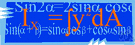Turing machine

(redirected from Deterministic Turing machine)
Also found in: Thesaurus, Acronyms, Encyclopedia.

Turing machine

n.
A hypothetical computing device capable of storing information and responding to computational questions, used in mathematical studies of computability.

[After Alan Mathison Turingwho conceived such a machine.]

Turing machine

n
(Computer Science) a hypothetical universal computing machine able to modify its original instructions by reading, erasing, or writing a new symbol on a moving tape of fixed length that acts as its program. The concept was instrumental in the early development of computer systems

Tu′ring machine`

(ˈtʊər ɪŋ, ˈtyʊər-)

n.
a hypothetical computing device used in mathematical studies of the computability of numbers and in theories of automata.
[after Alan M. Turing (1912–54), English mathematician, who described such a machine in 1936]
ThesaurusAntonymsRelated WordsSynonymsLegend:
 Noun 1Turing machine - a hypothetical computer with an infinitely long memory tapecomputer, computing device, computing machine, data processor, electronic computer, information processing system - a machine for performing calculations automatically
Translations
Turingův stroj
Turingov stroj
References in periodicals archive ?
(i) The family [PI] is polynomial uniform by Turing machine; that is, there exists a deterministic Turing machine constructing the system [product](n) for n [member of] N in polynomial time.
(k) the family [PI] is polynomially uniform with respect to Turing machines; that is, there exists a deterministic Turing machine working in polynomial time which constructs the system [product](e) with knowledge involving only the size of the problem X for every instance of X;
Therefore, there exists a deterministic Turing machine working in polynomial time which constructs the system with knowledge only of the number of nodes in the graph; that is, the family of P systems with d-division [product](n, e) is constructed in a uniform way.

Site: Follow: Share:
Open / Close